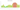# MDL

## Moldovan Leu

Moldova's official currency is the leu. The Moldovan leu is divided into 100 bani, just like the Romanian leu. The term "lion" is the source of the currency's name, which is derived from Romanian.

#### how has the value of the currency changed in the last year?## USD 1 = MDL 18.149

How much is10 US dollars worth inMoldovan lei?
At the current exchange rate, 10 US dollars is worth 181.49 Moldovan lei
How much is50 US dollars worth inMoldovan lei?
At the current exchange rate, 50 US dollars is worth 907.45 Moldovan lei
How much is100 US dollars worth inMoldovan lei?
At the current exchange rate, 100 US dollars is worth 1,814.89 Moldovan lei
How much is500 US dollars worth inMoldovan lei?
At the current exchange rate, 500 US dollars is worth 9,074.45 Moldovan lei
How much is2,000 US dollars worth inMoldovan lei?
At the current exchange rate, 2,000 US dollars is worth 36,297.81 Moldovan lei
How much is10 US dollars worth inMoldovan lei?
At the current exchange rate, 10 US dollars is worth 181.49 Moldovan lei
How much is50 US dollars worth inMoldovan lei?
At the current exchange rate, 50 US dollars is worth 907.45 Moldovan lei
How much is100 US dollars worth inMoldovan lei?
At the current exchange rate, 100 US dollars is worth 1,814.89 Moldovan lei
How much is500 US dollars worth inMoldovan lei?
At the current exchange rate, 500 US dollars is worth 9,074.45 Moldovan lei
How much is2,000 US dollars worth inMoldovan lei?
At the current exchange rate, 2,000 US dollars is worth 36,297.81 Moldovan lei